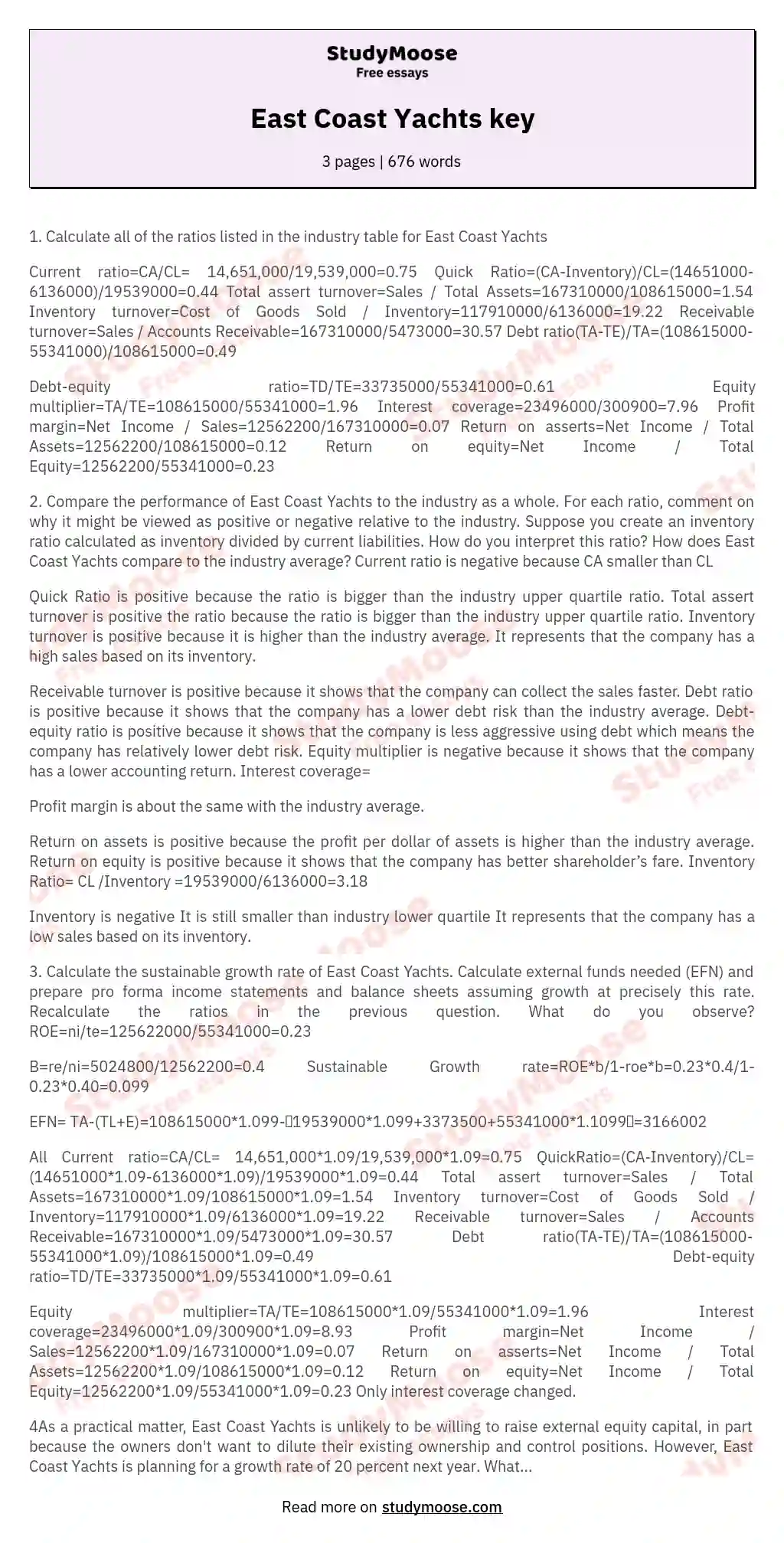# East Coast Yachts key

1. Calculate all of the ratios listed in the industry table for East Coast Yachts

Current ratio=CA/CL= 14,651,000/19,539,000=0.75
Quick Ratio=(CA-Inventory)/CL=(14651000-6136000)/19539000=0.44 Total assert turnover=Sales / Total Assets=167310000/108615000=1.54 Inventory turnover=Cost of Goods Sold / Inventory=117910000/6136000=19.22 Receivable turnover=Sales / Accounts Receivable=167310000/5473000=30.57 Debt ratio(TA-TE)/TA=(108615000-55341000)/108615000=0.49

Debt-equity ratio=TD/TE=33735000/55341000=0.61
Equity multiplier=TA/TE=108615000/55341000=1.96
Interest coverage=23496000/300900=7.96
Profit margin=Net Income / Sales=12562200/167310000=0.07
Return on asserts=Net Income / Total Assets=12562200/108615000=0.12 Return on equity=Net Income / Total Equity=12562200/55341000=0.23

2. Compare the performance of East Coast Yachts to the industry as a whole.

For each ratio, comment on why it might be viewed as positive or negative relative to the industry. Suppose you create an inventory ratio calculated as inventory divided by current liabilities. How do you interpret this ratio? How does East Coast Yachts compare to the industry average? Current ratio is negative because CA smaller than CL

Quick Ratio is positive because the ratio is bigger than the industry upper quartile ratio.

Get quality help nowDoctor JenniferVerified writer5 (893)

“ Thank you so much for accepting my assignment the night before it was due. I look forward to working with you moving forward ”+84 relevant experts are online

Total assert turnover is positive the ratio because the ratio is bigger than the industry upper quartile ratio. Inventory turnover is positive because it is higher than the industry average. It represents that the company has a high sales based on its inventory.

Receivable turnover is positive because it shows that the company can collect the sales faster. Debt ratio is positive because it shows that the company has a lower debt risk than the industry average. Debt-equity ratio is positive because it shows that the company is less aggressive using debt which means the company has relatively lower debt risk.

Get to Know The Price Estimate For Your Paper
Topic
Number of pages
Email Invalid email

You won’t be charged yet!

Equity multiplier is negative because it shows that the company has a lower accounting return. Interest
coverage=

Profit margin is about the same with the industry average.

Return on assets is positive because the profit per dollar of assets is higher than the industry average. Return on equity is positive because it shows that the company has better shareholder’s fare. Inventory Ratio= CL /Inventory =19539000/6136000=3.18

Inventory is negative It is still smaller than industry lower quartile It represents that the company has a low sales based on its inventory.

3. Calculate the sustainable growth rate of East Coast Yachts. Calculate external funds needed (EFN) and prepare pro forma income statements and balance sheets assuming growth at precisely this rate. Recalculate the ratios in the previous question. What do you observe? ROE=ni/te=125622000/55341000=0.23

B=re/ni=5024800/12562200=0.4
Sustainable Growth rate=ROE*b/1-roe*b=0.23*0.4/1-0.23*0.40=0.099

EFN= TA-(TL+E)=108615000*1.099-（19539000*1.099+3373500+55341000*1.1099）=3166002

All Current ratio=CA/CL= 14,651,000*1.09/19,539,000*1.09=0.75 QuickRatio=(CA-Inventory)/CL=(14651000*1.09-6136000*1.09)/19539000*1.09=0.44 Total assert turnover=Sales / Total Assets=167310000*1.09/108615000*1.09=1.54 Inventory turnover=Cost of Goods Sold / Inventory=117910000*1.09/6136000*1.09=19.22 Receivable turnover=Sales / Accounts Receivable=167310000*1.09/5473000*1.09=30.57 Debt ratio(TA-TE)/TA=(108615000-55341000*1.09)/108615000*1.09=0.49 Debt-equity ratio=TD/TE=33735000*1.09/55341000*1.09=0.61

Equity multiplier=TA/TE=108615000*1.09/55341000*1.09=1.96
Interest coverage=23496000*1.09/300900*1.09=8.93
Profit margin=Net Income / Sales=12562200*1.09/167310000*1.09=0.07 Return on
asserts=Net Income / Total Assets=12562200*1.09/108615000*1.09=0.12 Return on equity=Net Income / Total Equity=12562200*1.09/55341000*1.09=0.23 Only interest coverage changed.

4As a practical matter, East Coast Yachts is unlikely to be willing to raise external equity capital, in part because the owners don’t want to dilute their existing ownership and control positions. However, East Coast Yachts is planning for a growth rate of 20 percent next year. What are your conclusions and recommendations about the feasibility of East Coast’s expansion plans?

EFN= TA-(TL+E)=108615000*1.2-（19539000*1.2+3373500+55341000*1.2）=8753040

5. Most assets can be increased as a percentage of sales. For instance, cash can be increased by any amount. However, fixed assets often must be increased in specific amounts because it is impossible, as a practical matter, to buy part of a new plant or machine. In this case a company has a “staircase” or “lumpy” fixed cost structure. Assume that East Coast Yachts is currently producing at 100 percent Of capacity. As a result, to expand production, the company must set up an entirely new line at a cost of \$30 million. Calculate the new EFN with this assumption. What does this imply about capacity utilization for East Coast Yachts next year?

Depreciation percentage= \$5,460,000 / \$93,964,000= .0581
Pro forma depreciation=0.581*123964000=7203221

EFN= TA-(TL+E)=108615000*1.2+3000000 -（19539000*1.2+3373500+55341000*1.2=23004405 The fixed assets have increased faster than sales, so the capacity utilization for next year will decrease.